# GLAM2: Gapped Local Alignment of Motifs

Version 1056
glam2 -O glam2_example_output_files -M p At.faa

If you use this program in your research, please cite: MC Frith, NFW Saunders, B Kobe, TL Bailey, "Discovering sequence motifs with arbitrary insertions and deletions", PLoS Computational Biology, 4(5):e1000071, 2008.[full text]

## Best Motif Found:

NAME START SITES END STRAND MARGINAL SCORE
At1g01140.1_ 116 E D E A R R Y F Q Q L I N A V D Y C H S . . . R G V Y H R D L K P E N L I L D A N G V L . . . . . . . . K V S D F G L S A 165 + 120.
At1g01140.2_ 116 E D E A R R Y F Q Q L I N A V D Y C H S . . . R G V Y H R D L K P E N L I L D A N G V L . . . . . . . . K V S D F G L S A 165 + 120.
At1g01140.3_ 116 E D E A R R Y F Q Q L I N A V D Y C H S . . . R G V Y H R D L K P E N L I L D A N G V L . . . . . . . . K V S D F G L S A 165 + 120.
At1g01450.1_ 123 L P V A V D L M L Q I A L G M E Y L H S . . . K R I Y H G E L N P S N I L V K P R S N Q S G D G Y L L G K I F G F G L N S 180 + 44.0
At1g01540.1_ 251 W D I R M N I I L G M A K G L A Y L H E G L E P K V V H R D I K S S N I L L D R Q W N A . . . . . . . . K V S D F G L A K 303 + 106.
At1g01540.2_ 251 W D I R M N I I L G M A K G L A Y L H E G L E P K V V H R D I K S S N I L L D R Q W N A . . . . . . . . K V S D F G L A K 303 + 106.
At1g01560.1_ 140 D D H S R F F L Y Q L L R G L K Y V H S . . . A N V L H R D L K P S N L L L N A N C D L . . . . . . . . K I G D F G L A R 189 + 84.9
At1g01740.1_ 151 W A M R L R V A L Y I S E A L E Y C S N N G . H T L Y H . D L N A Y R V L F D E E C N P . . . . . . . . R L S T F G L M K 201 + 12.2
At1g02970.1_ 346 E R E I L V I M H Q I A K A L H F V H E . . . K G I A H L D V K P D N I Y I K . N G V C . . . . . . . . K L G D F G C A T 394 + 69.3
At1g03740.1_ 311 E P Q V K C Y M R Q L L S G L E H C H S . . . R G V L H R D I K G S N L L I D S K G V L . . . . . . . . K I A D F G L A T 360 + 100.
At1g03920.1_ 234 E D E A K F Y I A E S V L A I E S I H N . . . R N Y I H R D I K P D N L L L D R Y G H L . . . . . . . . R L S D F G L C K 283 + 79.2
At1g03930.1_ 102 L K T V L M L A D Q L I N R V E F M H T . . . R G F L H R D I K P D N F L M G L G R K A N Q V . . . . . Y I I D F G L G K 154 + 86.4
At1g04210.1_ 901 M D L A L S I A R D I S G A L M E L H S . . . K D I I H R D I K S E N V L I D . L D N Q S A N G E P I V K L C D F D R A V 957 + 49.6
At1g04440.1_ 102 L K T V L M L A D Q M I N R V E Y M H V . . . K G F L H R D I K P D N F L M G L G R K A N Q V . . . . . Y I I D Y G L A K 154 + 81.5
At1g04700.1_ 869 R R K K L M I T L D S A F G M E Y L H M . . . K N I V H F D L K C D N L L V N L R D P Q R P I C . . . . K V G D F G L S R 922 + 65.3
At1g05100.1_ 104 E A R V V K Y T R Q I L L G L E Y I H N S . . K G I A H C D I K G S N V L V G E N G E A . . . . . . . . K I A D F G C A K 154 + 90.7
At1g05700.1_ 655 W R Q R L Q I A L D A A Q G L E Y L H C G C K P P I V H R D V K T S N I L L N E K N R A . . . . . . . . K L A D F G L S R 707 + 78.3
At1g06390.1_ 168 I F Y V K L Y T Y Q I F R G L A Y I H T V . . P G V C H R D V K P Q N L L V D P L T H Q V . . . . . . . K L C D F G S A K 219 + 105.
At1g06390.2_ 168 I F Y V K L Y T Y Q I F R G L A Y I H T V . . P G V C H R D V K P Q N L L V D P L T H Q V . . . . . . . K L C D F G S A K 219 + 105.
 logo with ssc Score: 1277.07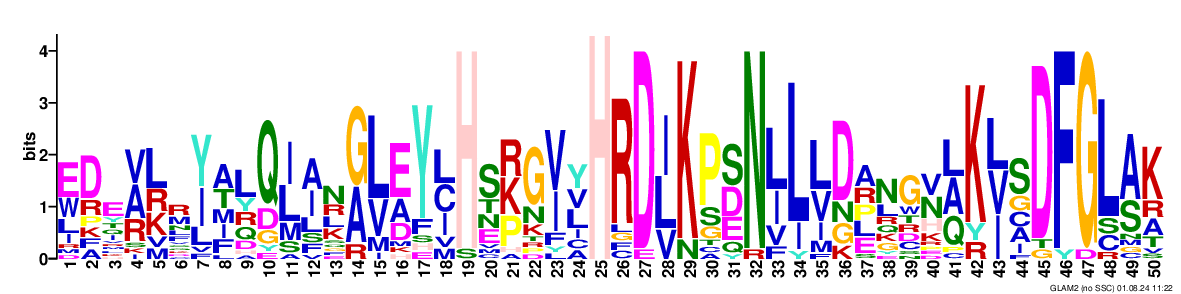against sequence databases using GLAM2SCAN.

Regular Expression for Motif: [EW]DE[ARV][KRL]R[YI][AT]LQ[IL][AI]N[AG][LV][AE]Y[ICL]HS.{0,3}[PKR]G[IV][LVY]HR?D[ILV]KP[SED]N[IL]L[LV]DA?NG[NV][QAL].{0,8}K[ILV]SDFGL[AS]K

## Replicates:

Check that at least some of these are similar to the best motif found.
If not, there may well be an even better motif.
Click here to with double the "maximum number of iterations without improvement" to find it.

 logo with ssc Score: 1271.22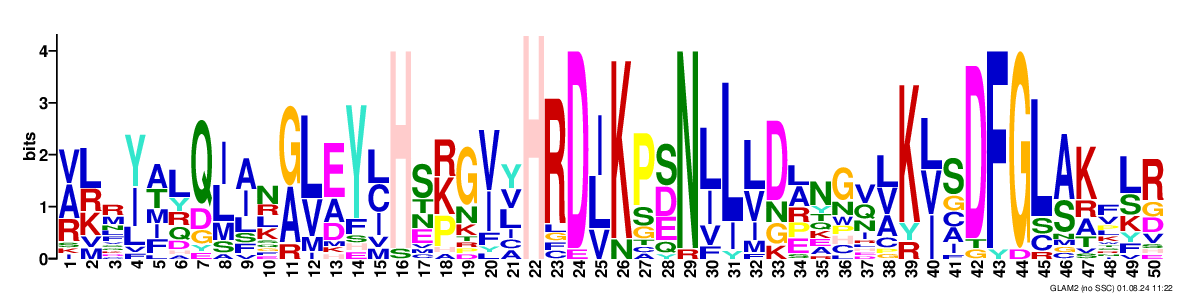against sequence databases using GLAM2SCAN.

Regular Expression for Motif: [ARV][KRL]R[YI][AT]LQ[IL][AI]N[AG][LV][AE]Y[ICL]HS.{0,3}[PKR]G[IV][LVY]HR?D[ILV]KP[SED]N[IL]L[LV]D[AL]?.{0,8}NG[QV][ALV]K[ILV]SDFGL[AS]KF[LS]R
 logo with ssc Score: 1250.03against sequence databases using GLAM2SCAN.

Regular Expression for Motif: [KRL]R[YI][AT]LQ[IL][AI]N[AG][LV][AE]Y[ICL]H.{0,3}S[PKR]G[IV][LVY]HR?D[ILV]KP[SED]N[IL]L[LV]D[AL]?.{0,8}[KN]G[NV]Q?[ALV]K[ILV]SDFGL[AS]KF[LS]R
 logo with ssc Score: 946.511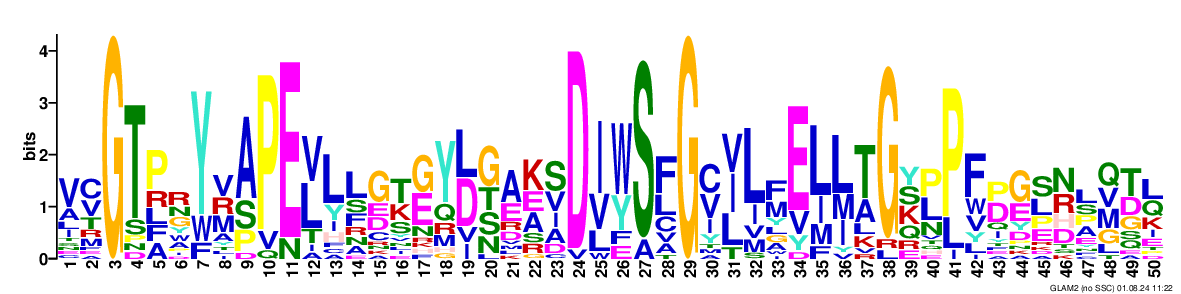against sequence databases using GLAM2SCAN.

Regular Expression for Motif: V[CV]G?TPRY[MRV][AS]PE?[LV]?LLG.{0,9}[KT][EG]Y[DL]?[STG][AE][AEK][SV]D[IV][YW]S[FL]G[CV][ILV]L[FM]E[IL][IML][AT]G[KSY][LP]P?F[DP][EG][LS][NR]?L[MQV][DT][LQ]
 logo with ssc Score: 922.481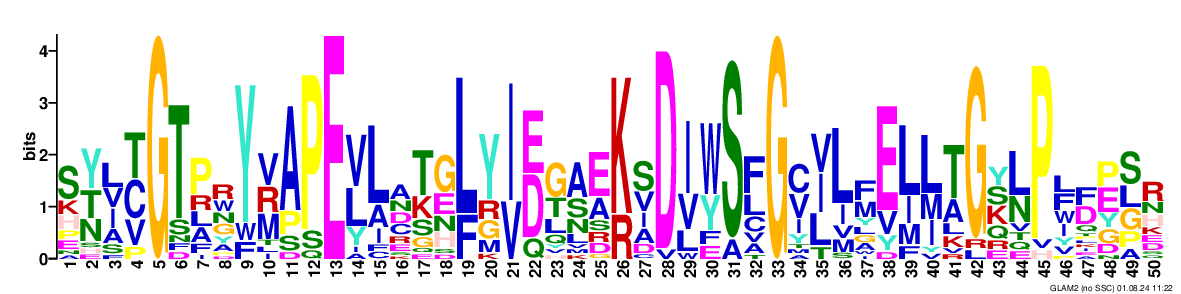against sequence databases using GLAM2SCAN.

Regular Expression for Motif: S[NTY][ILV][CTV].{0,5}G?TPRY[MRV]?APE?[LV]L?.{0,8}A[KT][EG][LF]?Y[IV]?[ED]?[GT][AS][AE][KR]?[SV]D[IV][YW]S[FL]G[CV][ILV]L[FM]E[IL][IML][AT]G[KSY][LN]?P[FL][DF][EP][SLG]R
 logo with ssc Score: 887.989against sequence databases using GLAM2SCAN.

Regular Expression for Motif: S[TY][ILV][CTV].{0,5}G?TPR.{0,2}Y[MRV]APE[LV]L.?[GT][AGK][GS]Y?[YDN]?[ETG].{0,9}[AEK][SV]D[IV][YW]S[FL]G[CV][ILV]L[FM]E[IL][IML][AT]G[KSY][LN]?P[IYL]?F[DP][EG]S[NR]L[MQV]
 logo with ssc Score: 848.504against sequence databases using GLAM2SCAN.

Regular Expression for Motif: [LV][LST]Y.[AV]?[CV]G?T[LP].Y[MRV]AP?E?[LVY]L.[GT][AK]?G.{0,8}H[LY][TG][AE]?[AKE][SV]D[IV][YWF]SFG[CV][ILV]LFE[IL][IML][AT]G?[KY][LN]?P[ILF]?[DF][EP][SG][LR]
 logo with ssc Score: 680.611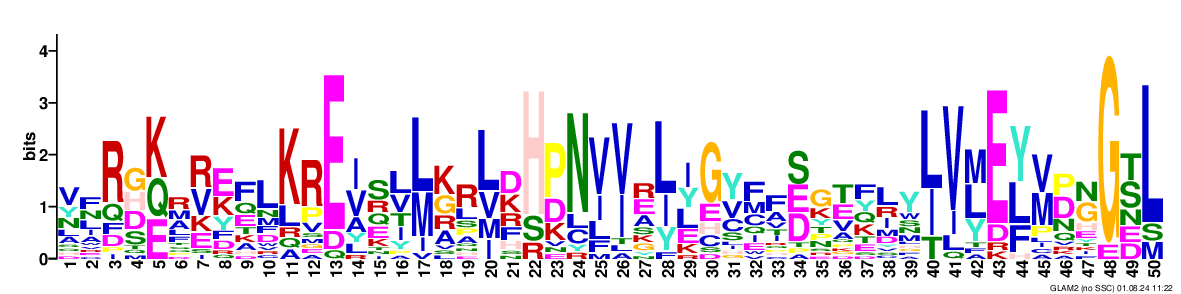against sequence databases using GLAM2SCAN.

Regular Expression for Motif: VFR[DGH][QKE]?R[RV][EK]FLK?RE[IV]S[LV][ML][GK]R[LV].{0,1}[DK]H[DP]N[IV][IV][ER][IL]?[ILY]G[VY]FF[EDS]G[ET].{0,13}FLY[IL]V[MLY]E[YLF][MV][DP][GN]G?[ST]L
 logo with ssc Score: 383.331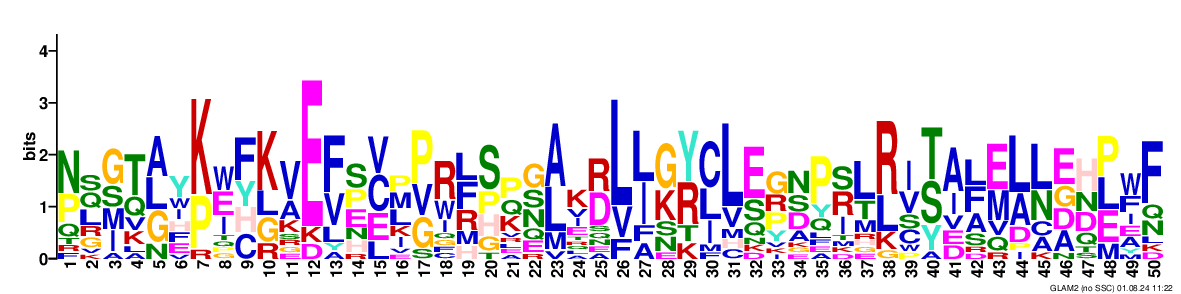against sequence databases using GLAM2SCAN.

Regular Expression for Motif: [NP][LQS][ISMG][QT][ALG]Y[PK].{0,3}[EIW][YHCF]?[KLG][AV].{0,2}E?[VF][EPS][CEV]?[MLP][PGV]?[RW].{0,2}[RLF][HSP][KQP][GNS][AL]K[DR][LV][IL][KG][YR][ICL]L?.{0,1}[ES][GPR][DNS]P[ISR][LT][RL][IV]?[YST].{0,1}[IA][AFL][ME][AL][LN][AEDG][DHN][PEL][FW]F
 logo with ssc Score: 281.318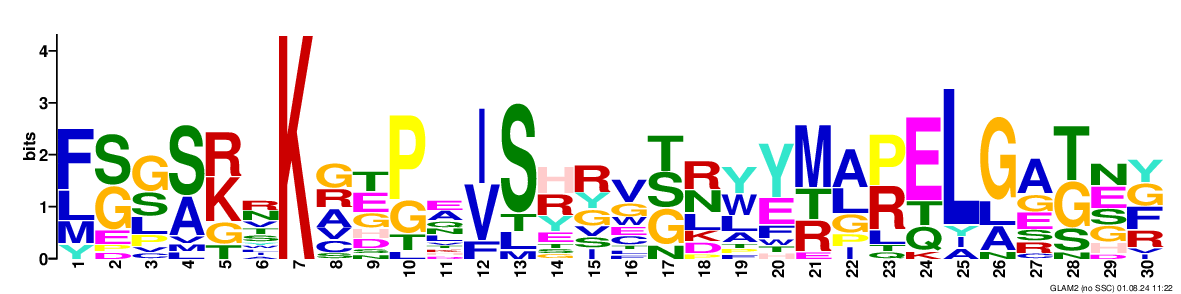against sequence databases using GLAM2SCAN.

Regular Expression for Motif: [LF][SG][GS][AS][KR]..{0,2}K?[AGR]?[ET][PG].[IV]?S[HR].{0,5}RV[STG][NR][WY][EY][RMT][AL][PR]EL?.{0,2}GA[TG][EN][GFY]